Filters
Size

# Tees + Cubans

Filters

Selected Filters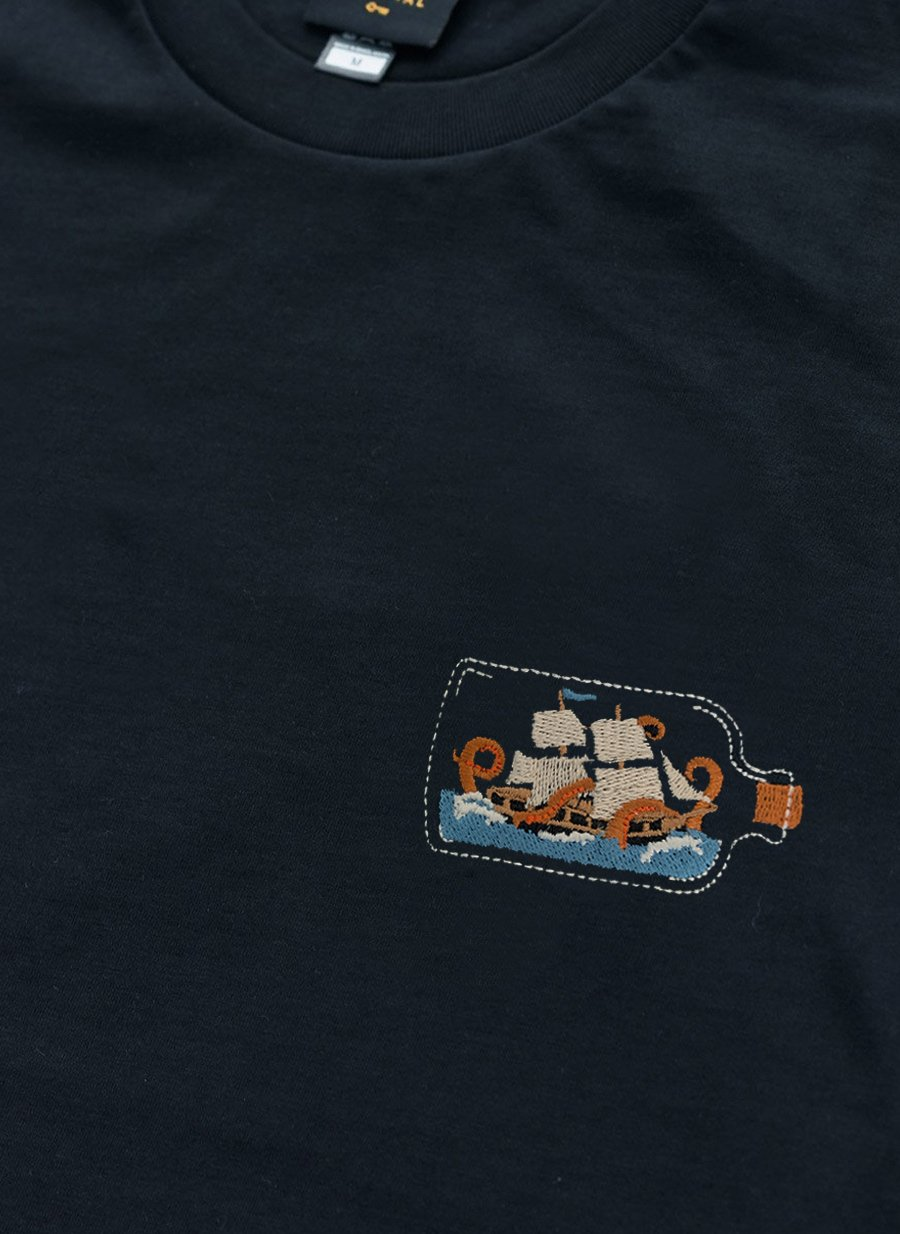• XS
• S
• M
• L
• XL
• XXL
• Select Size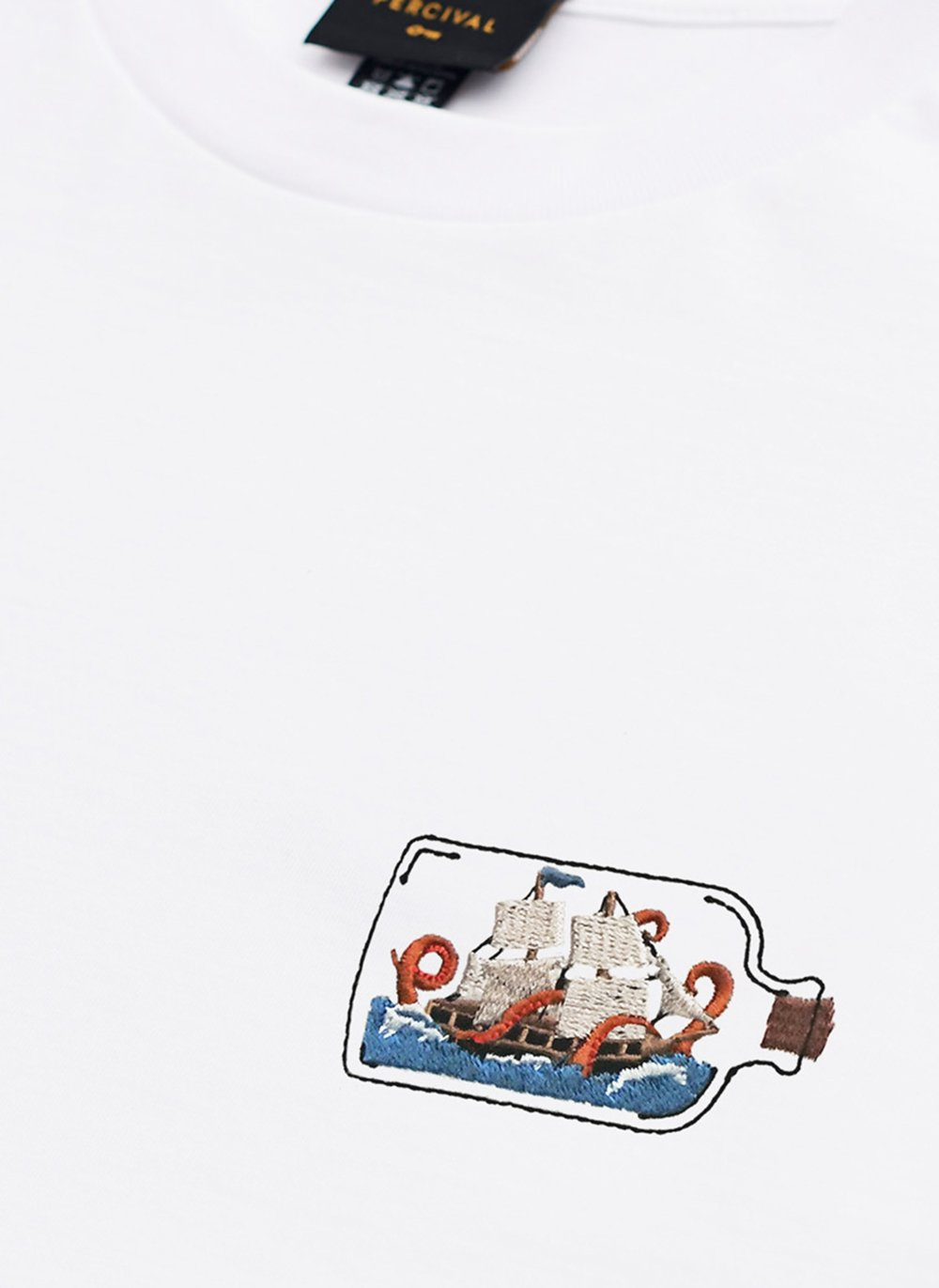• XS
• S
• M
• L
• XL
• XXL
• Select Size• XS
• S
• M
• L
• XL
• XXL
• Select Size• XS
• S
• M
• L
• XL
• XXL
• Select Size• XS
• S
• M
• L
• XL
• XXL
• Select Size
Sold Out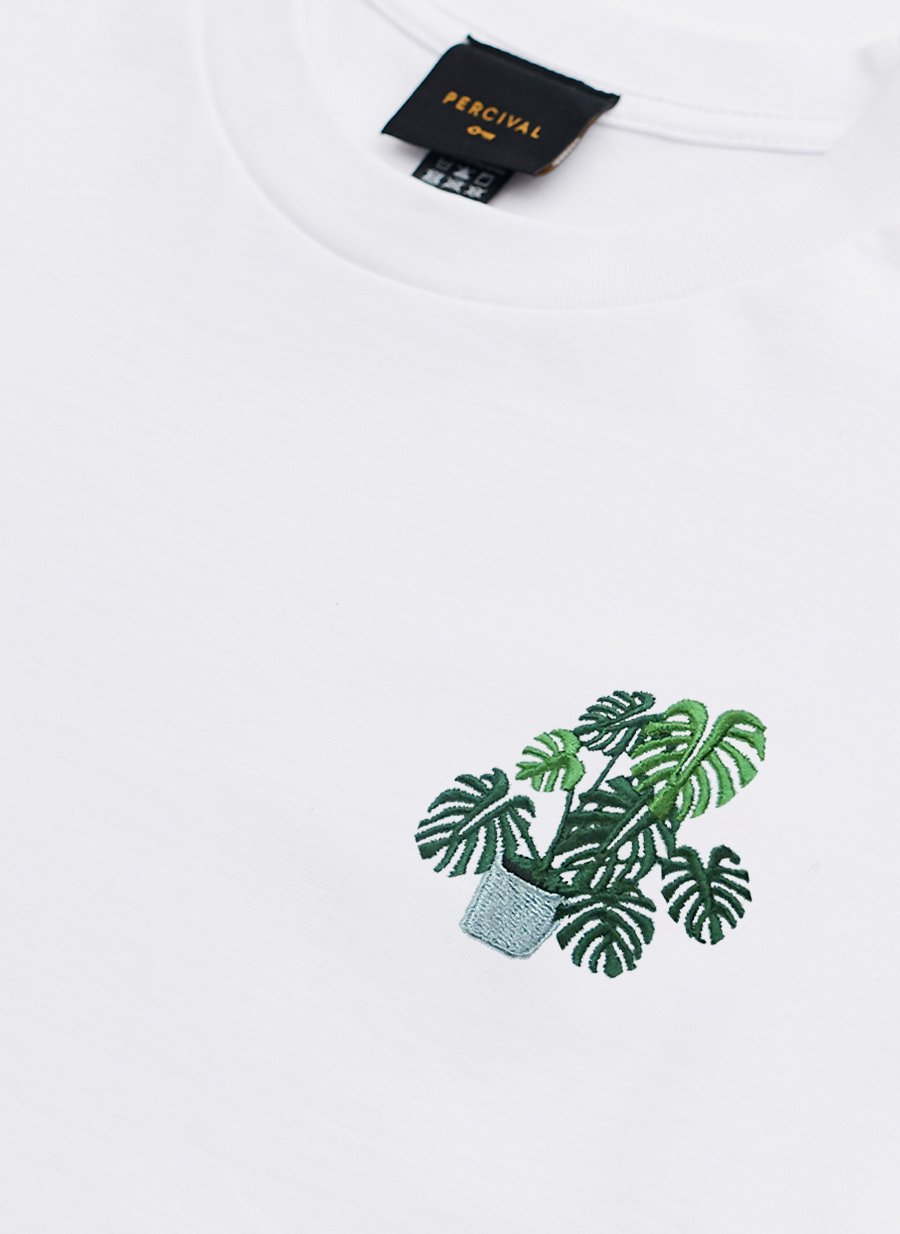• XS
• S
• M
• L
• XL
• XXL
• Select Size• XS
• S
• M
• L
• XL
• XXL
• Select Size
Running Low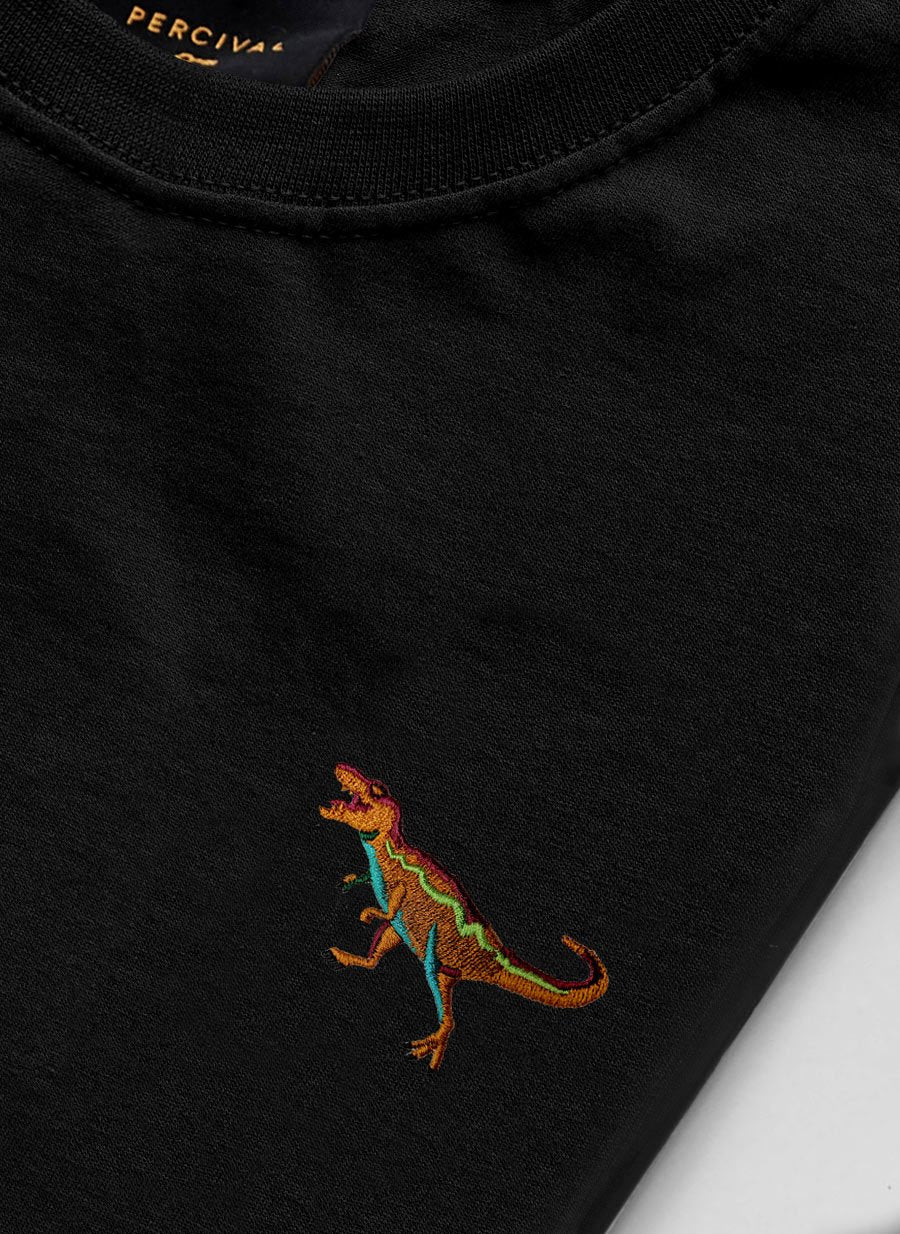• XS
• S
• M
• L
• XL
• XXL
• Select Size• XS
• S
• M
• L
• XL
• XXL
• Select Size• XS
• S
• M
• L
• XL
• XXL
• Select Size• XS
• S
• M
• L
• XL
• XXL
• Select Size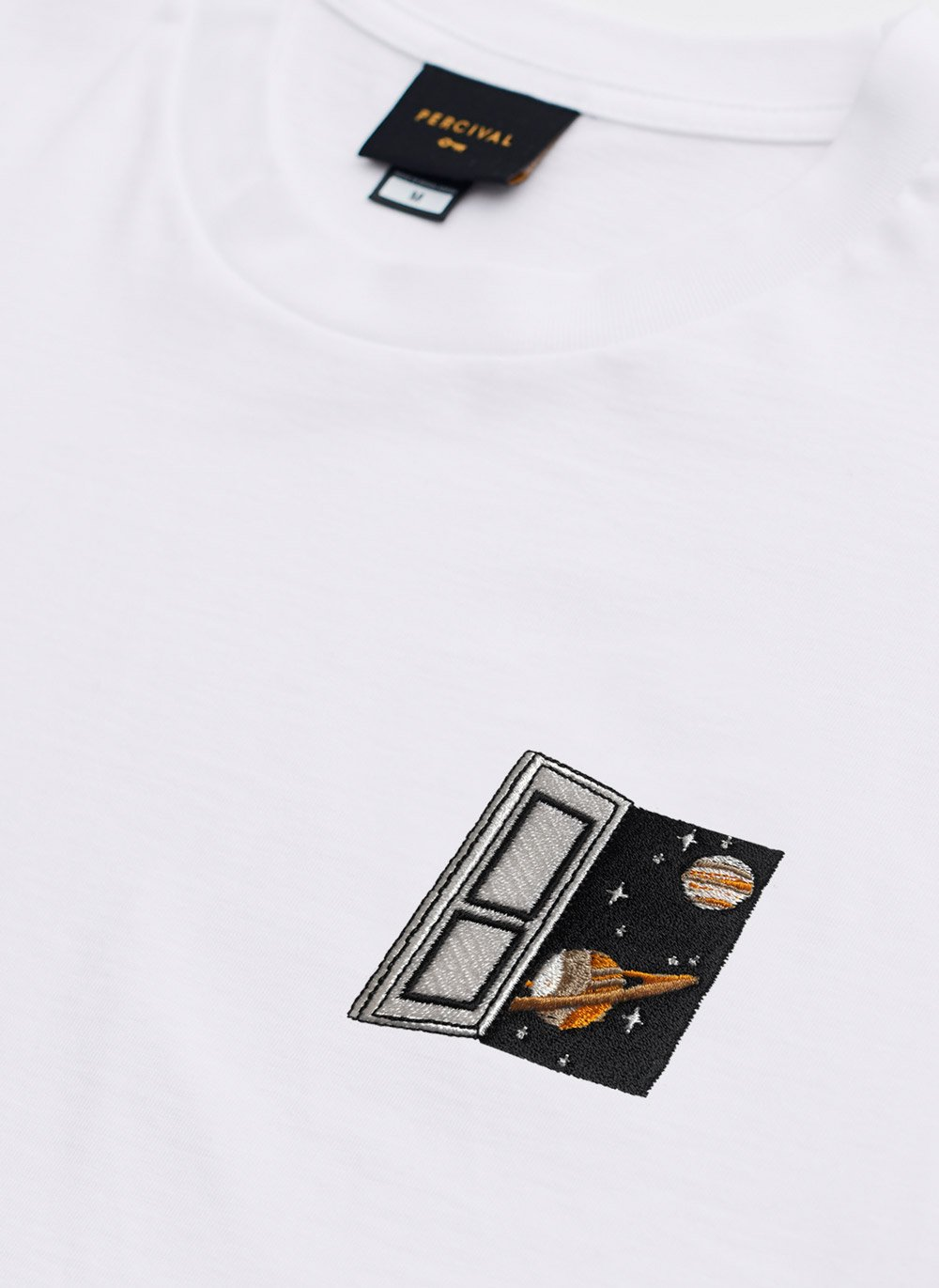• XS
• S
• M
• L
• XL
• XXL
• Select Size